Question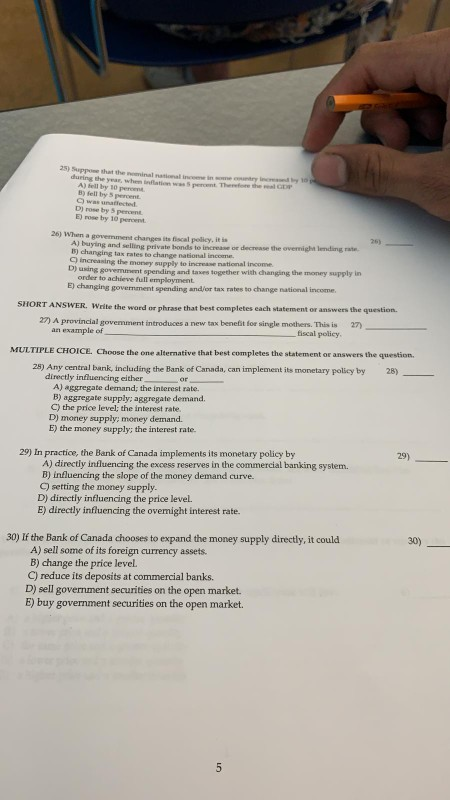its mcq and short question answer.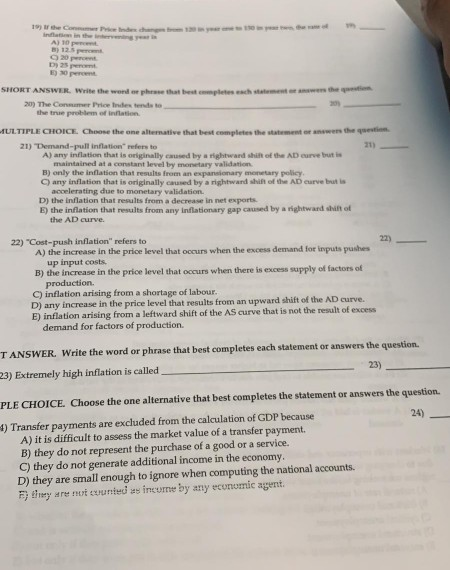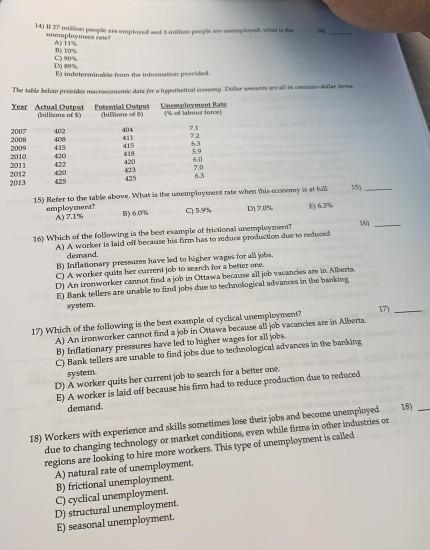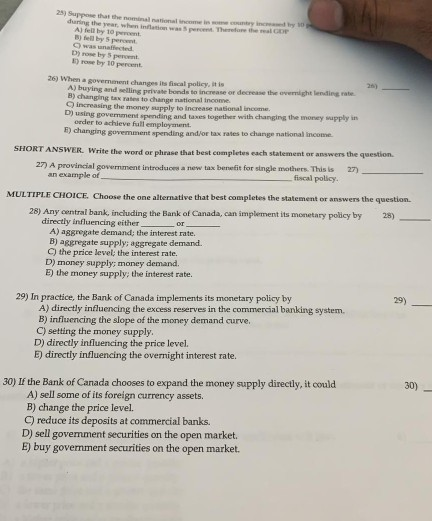1) Solution: 25%

Explanation: The inflation rate between Year 1 and Year 2 is the percentage change in the price indices during these two years

(150 - 120) / 120 * 100 = 30/120 * 100 = 25%

2) Solution: overstate

Explanation: Due to substitution bias, new product bias and quality bias CPI tends overstate the inflation

3) Solution: rose by 5 percent

Explanation: Real National Income = Nominal national income - Inflation = 10% - 5 % = 5%

4) Solution: changing the government spending and/or tax to change national income

Explanation: In the fiscal policy the government adjusts its tax rates and spending levels to influence a country's economy.

5) Solution: Expansionary

Explanation: Expansionary fiscal policy is increased tax benefits or government spending

As per policy we have to answer first four questions

#### Earn Coins

Coins can be redeemed for fabulous gifts.

Similar Homework Help Questions
• ### MULTIPLE CHOICE. Choose the one alternative that best completes the statement or answers the question. 21)...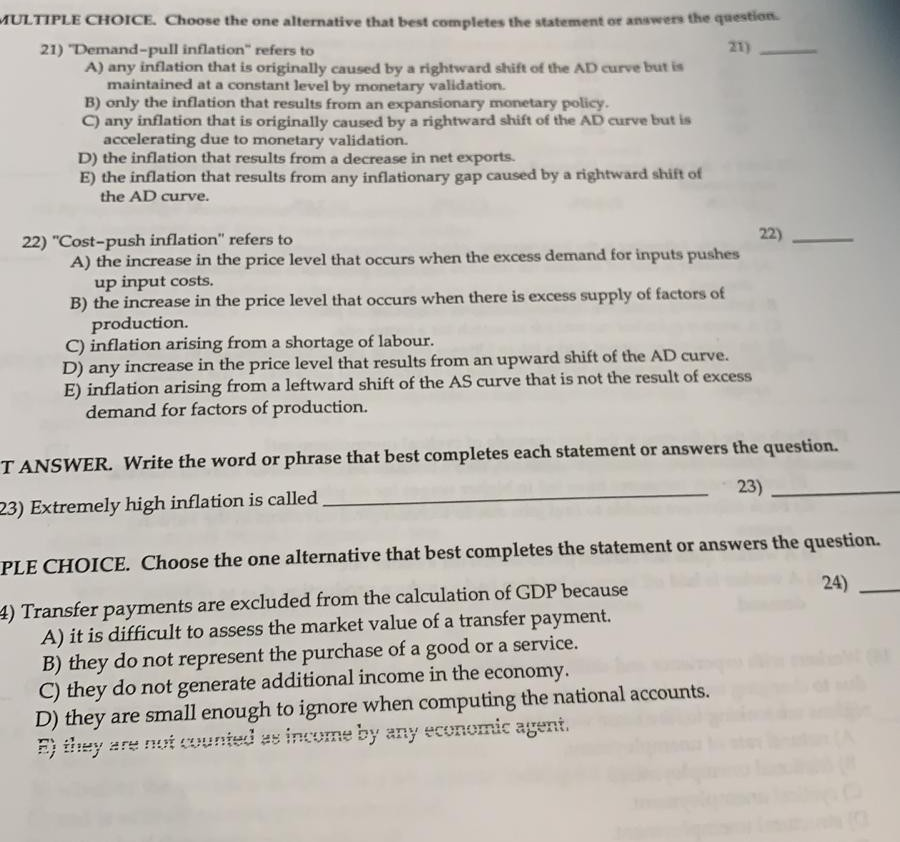MULTIPLE CHOICE. Choose the one alternative that best completes the statement or answers the question. 21) 21) "Demand-pull inflation" refers to A) any inflation that is originally caused by a rightward shift of the AD curve but is maintained at a constant level by monetary validation. B) only the inflation that results from an expansionary monetary policy. C) any inflation that is originally caused by a rightward shift of the AD curve but is accelerating due to monetary validation. D)...

• ### 2. Suppose the economy is in long-run equilibrium, with real GDP at \$19 trillion and the unemployment rate at 5%. Now assume that the central bank unexpectedly decreases money supply by 6%. a) Illust...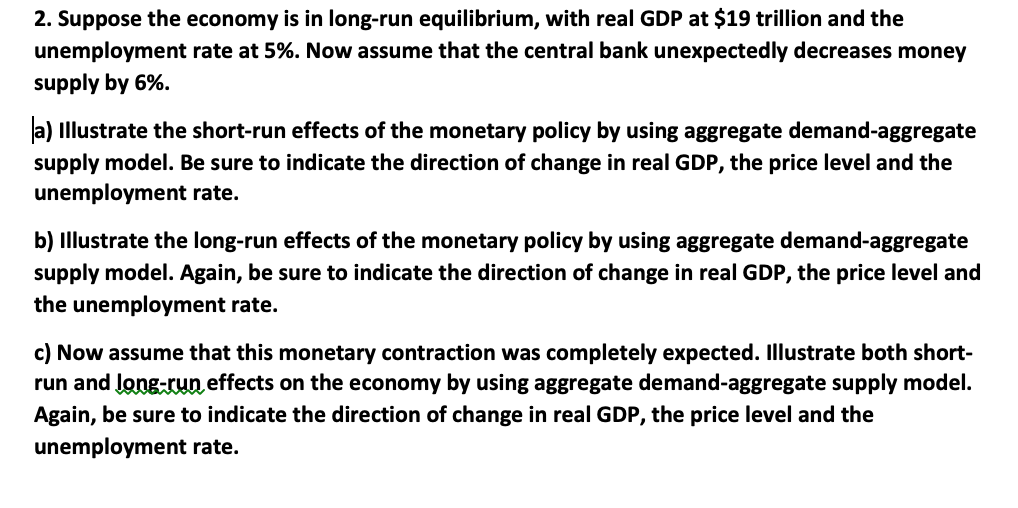2. Suppose the economy is in long-run equilibrium, with real GDP at \$19 trillion and the unemployment rate at 5%. Now assume that the central bank unexpectedly decreases money supply by 6%. a) Illustrate the short-run effects of the monetary policy by using aggregate demand-aggregate supply model. Be sure to indicate the direction of change in real GDP, the price level and the unemployment rate. b) Illustrate the long-run effects of the monetary policy by using aggregate demand-aggregate supply model....

• ### 1) of the Central Bank of Kuwait puts in place an expansionary monetary policy, its decision...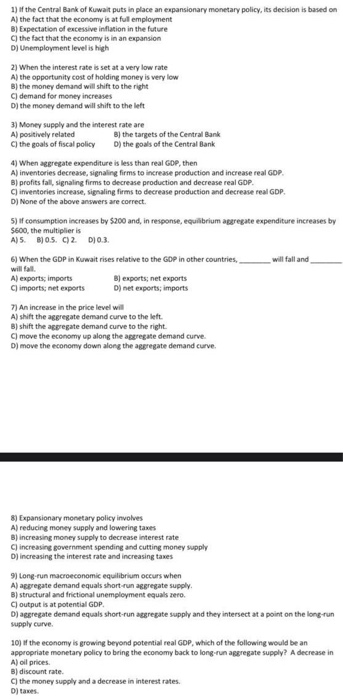1) of the Central Bank of Kuwait puts in place an expansionary monetary policy, its decision is based on A) the fact that the economy is at ful employment B) Expectation of excessive inflation in the future C) the fact that the economy is in an expansion D) Unemployment level is high 2) When the interest rate is set at a very low rate A) the opportunity cost of holding money is very low B) the money demand will shift...

• ### Macroeconomic Multiple Choice Questions Answer All 10 Questions* 1) If the Central Bank of Kuwait puts...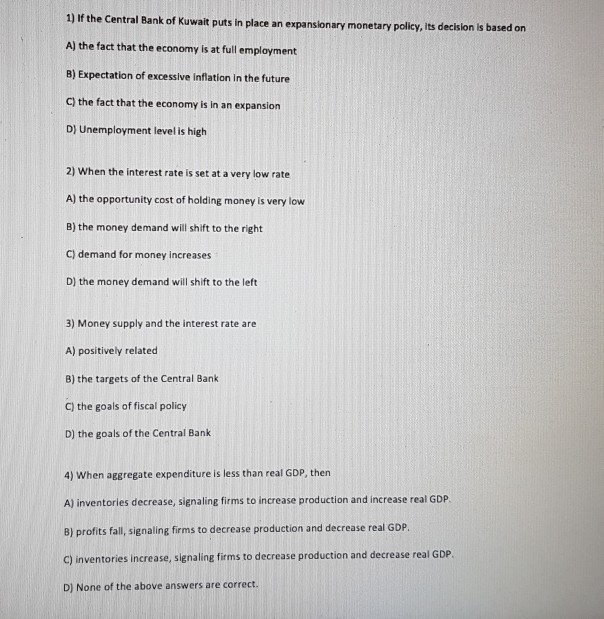Macroeconomic Multiple Choice Questions Answer All 10 Questions* 1) If the Central Bank of Kuwait puts in place an expansionary monetary policy, its decision is based on A) the fact that the economy is at full employment B) Expectation of excessive inflation in the future C) the fact that the economy is in an expansion D) Unemployment level is high 2) When the interest rate is set at a very low rate A) the opportunity cost of holding money is...

• ### An increase in the price level will A) shift the aggregate demand curve to the left.

7) An increase in the price level will A) shift the aggregate demand curve to the left. B) shift the aggregate demand curve to the right. C) move the economy up along the aggregate demand curve. D) move the economy down along the aggregate demand curve. 8) Expansionary monetary policy involves A) reducing money supply and lowering taxes B) increasing money supply to decrease interest rate C) increasing government spending and cutting money supply D) increasing the interest rate and increasing taxes 9) Long-run macroeconomic equilibrium occurs when A) aggregate demand...

• ### 2. Phillips Curve. An economy has the following functions for its short run aggregate supply (SRAS),...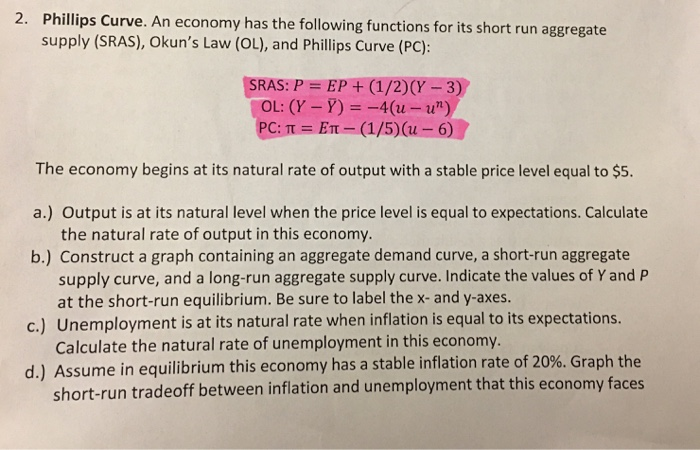2. Phillips Curve. An economy has the following functions for its short run aggregate supply (SRAS), Okun's Law (OL), and Phillips Curve (PC): SRAS: P = EP + (1/2)(y - 3) OL: (Y-Y) = -4(u-u") PC:T = ET - (1/5)( - 6) The economy begins at its natural rate of output with a stable price level equal to \$5. a.) Output is at its natural level when the price level is equal to expectations. Calculate the natural rate of output...

• ### Economics: 1) Why is it possible to change real economic factors in the short run simply...

Economics: 1) Why is it possible to change real economic factors in the short run simply by printing and distributing more money? 2) Explain why a stable 5% inflation rate can be preferable to one that averages 4% but varies between 1-7% regularly. 3) Explain the difference between active and passive monetary policy. 4) Suppose the economy is in long-run equilibrium, with real GDP at \$16 trillion and the unemployment rate at 5%, Now assume that the central bank unexpectedly...

• ### 1.The Aggregate Supply curve shows which of the following relationships: the inverse relationship between the price...

1.The Aggregate Supply curve shows which of the following relationships: the inverse relationship between the price level and real income the positive relationship between the price level for goods and domestic output the combinations of income and the interest rate for which the demand for money equals the money supply 2.When a central bank buys long-dated government securities, it is most likely trying to do which of the following? reduce consumption and borrowing to lower inflation and growth reduce the...

• ### What determines the magnitude of the changes in price level when central bank takes monetary policy...

What determines the magnitude of the changes in price level when central bank takes monetary policy measures that leads to a change in the aggregate demand? a. Changes in the money supply b. Slope of the aggregate supply curve c. Rate of change of interest rate d. Total money supply in the economy

• ### Money Demand According to Liquidity Preference Theery, why is the Money Demand curve downwaed sloping? a...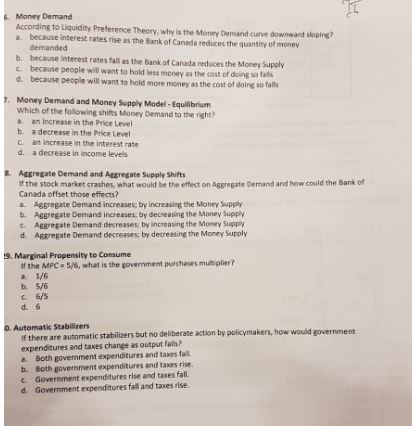Money Demand According to Liquidity Preference Theery, why is the Money Demand curve downwaed sloping? a because interest rates rise as the Bank of Canada reduces the quantity of money demanded b. because interest rates fall as the Bank of Canada reduces the Money Supply c because people will want to hold less money as the cost of doing so fals d. because people will want to hold more money as the cost of doing so falls Money Demand and...